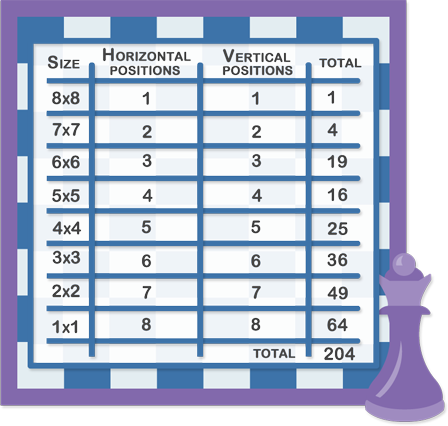# IQ Test Labs

Brainteasers > Pictures > Squares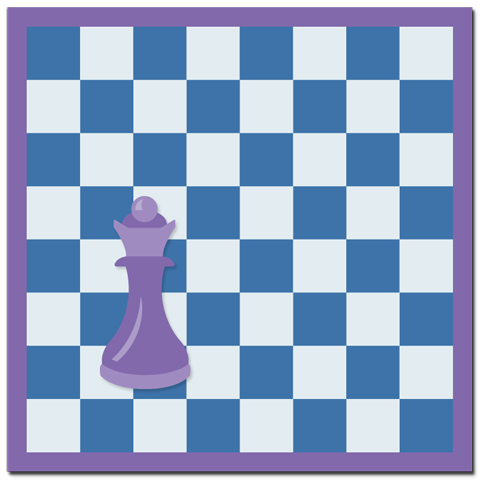Spot the squares within the squares! There are squares of all sizes in the figures.

• Middle squares: Dont' forget to add the middle squares!
• Circle squares: These squares have a common core.
• Chessboard squares: How many squares are there in a chessboard?
• 1. Middle squares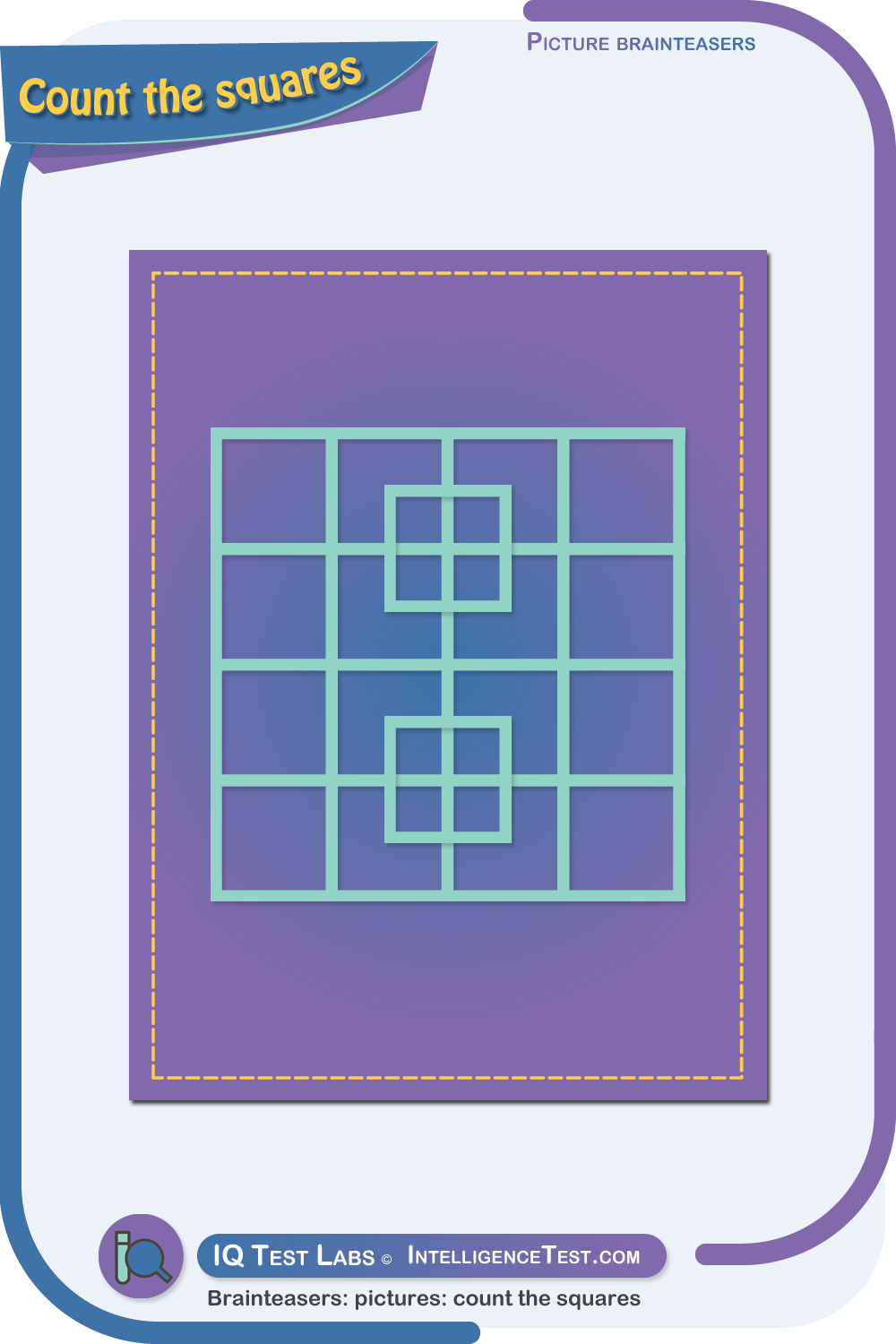There are fourty squares.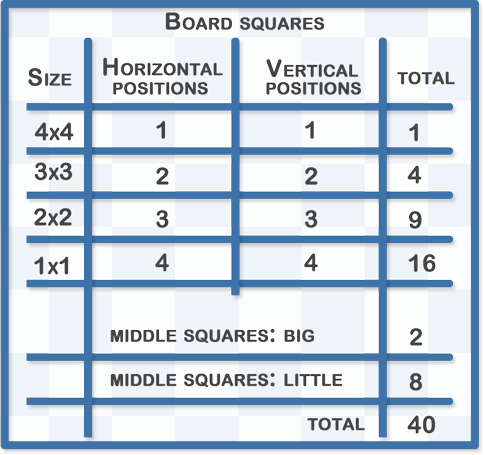2. Circle squares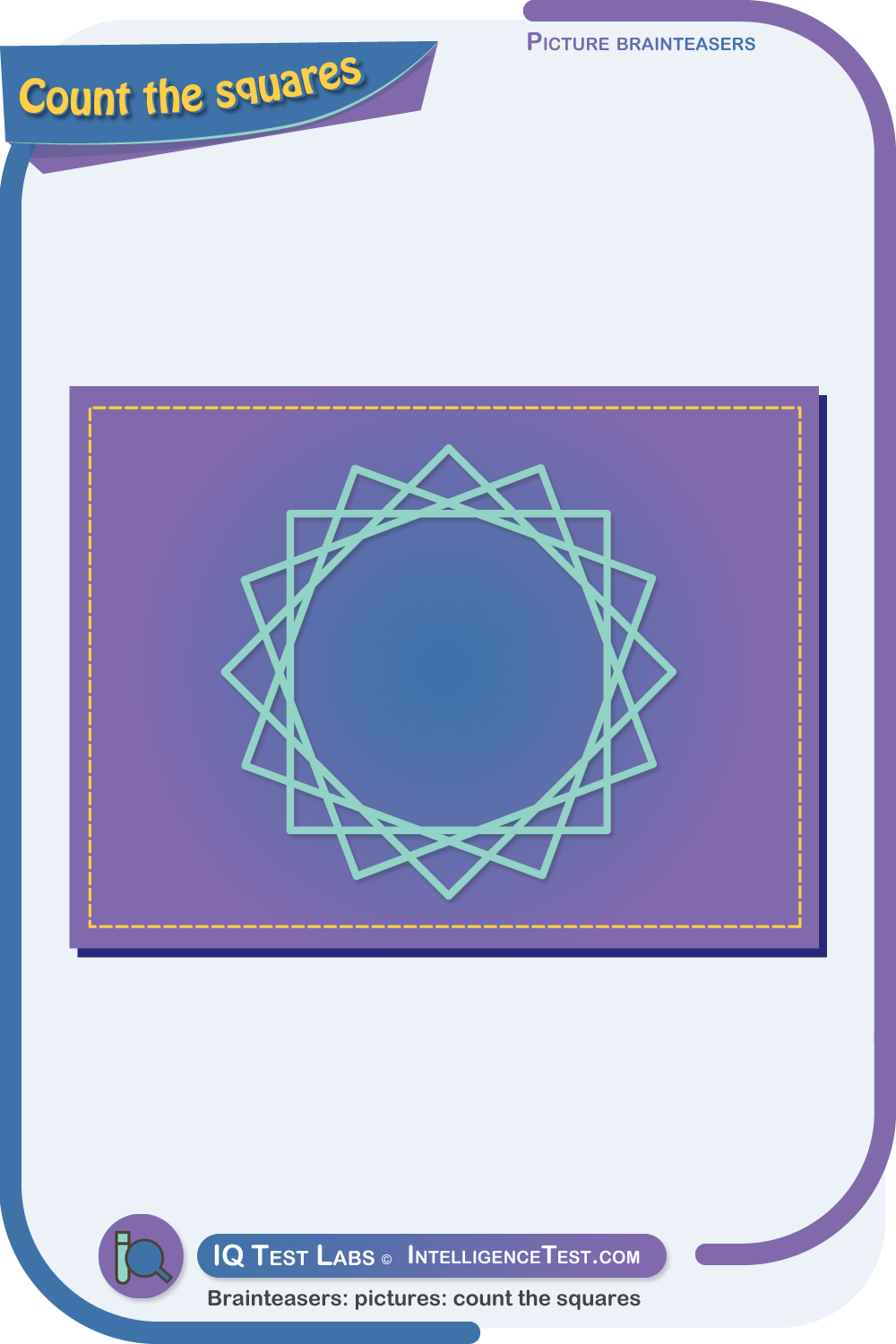There are four squares.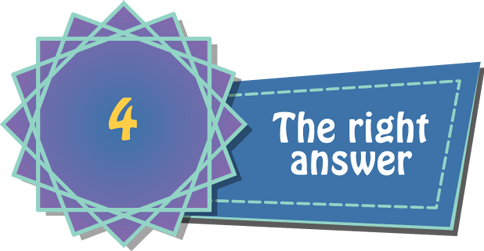3. Chessboard squares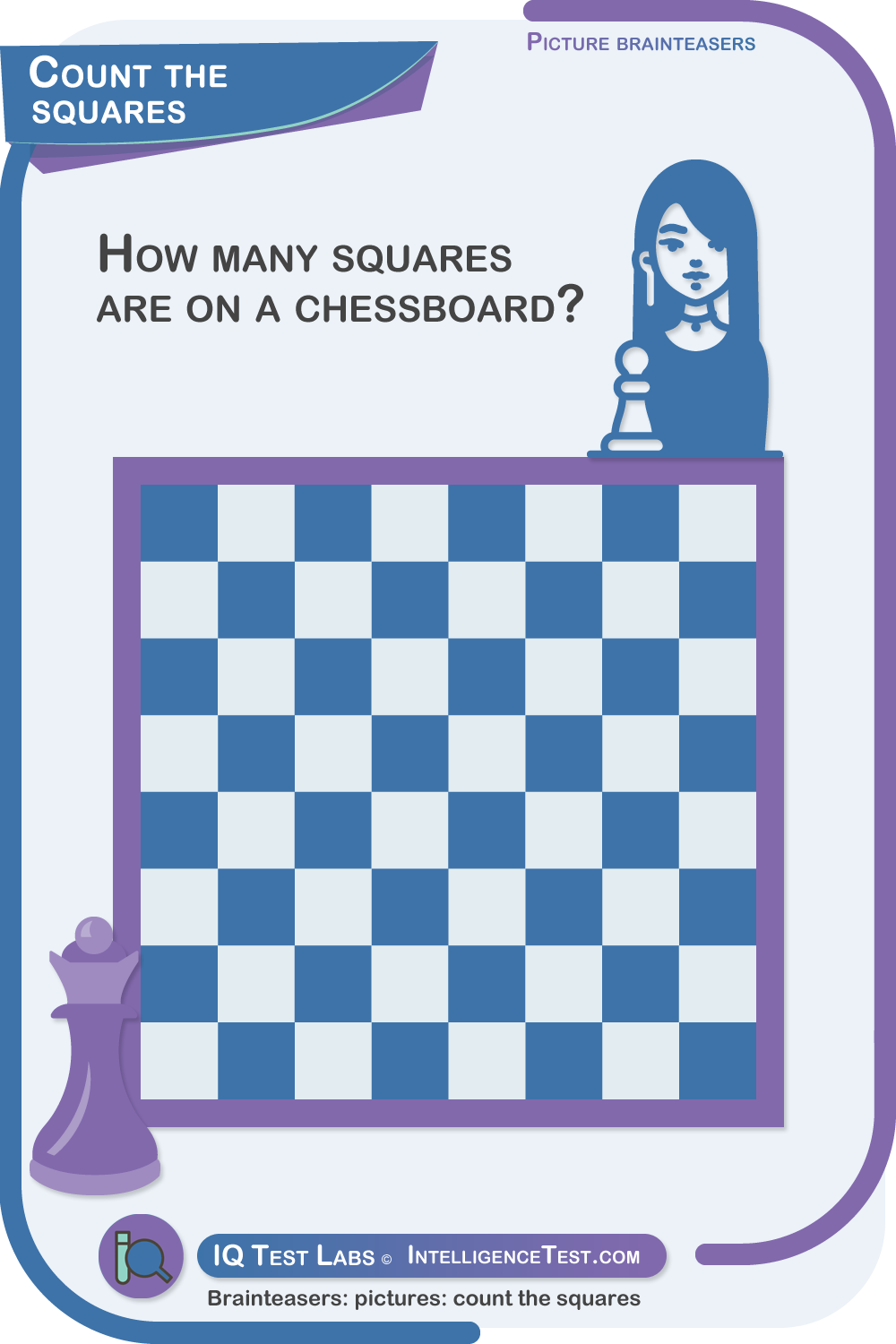There are two hundred and four squares.